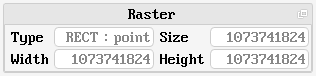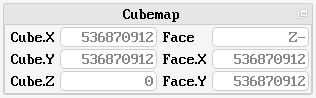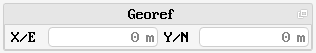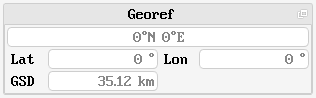# Map Coordinates

## GUI component

The Tinman 3D SDK contains a geo-referencing API which can be used to translate between various coordinate systems (see `CoordinateSystem` API).

The Map Coordinates GUI component displays map coordinates in different coordinate systems, dependant on the kind of geo-reference that is associated with the underlying map.The Raster section shows the properties of the underlying raster.

### Type

The raster type can be one of the following:

• RECT : point
A rectangular raster with sample-is-point semantic.
This type usually represents local digital elevation models.

• RECT : area A rectangular raster with sample-is-area semantic.
This type usually represents local satellite imagery.

• CUBE : point
A cubemap raster with sample-is-point semantic.
This type usually represents global digital elevation models.

• CUBE : area
A cubemap raster with sample-is-area semantic.
This type usually represents global satellite imagery.

### Size / Width / Height

Rectangular rasters can have indepedent values for width and height. The raster size is the smallest power of two that is greater than or equal to the maximum of width and height.

Width, height and size always refer to the edge length of the raster. For sample-is-area, the edge length corresponds to the number of samples; for sample-is-point, the edge length is equal to the number of samples minus one.The Cubemap section shows the coordinates in cubemap space. Rectangular rasters are expressed in cubemap space by using the negative-Z face only.

For details, please refer to the `Cubemap` API.

### Cube X/Y/Z

The cubemap coordinates along the cubemap X-, Y- and Z-axis. Cubemap coordinates are unique, i.e. each sample is referred to by a distinct set of cubemap coordinates.

### Face

The cubemap face (X-, X+, Y-, Y+, Z-, Z+) that contains the raster sample. Edge and corner samples are contained by two resp. three cubemap faces.

### Face X/Y

The cubemap face coordinates along the X- and Y-axes of the cubemap face. Cubemap face coordinates are not unique; the sample sample can be referred to by different sets of cubemap face coordinates.If the geo-reference includes a definition of a projected two-dimensional map (e.g. Mercator), the Georef section shows the map coordinates, which are usually termed Easting and Northing.

Map coordinates are not necessarily referenced to a geographic coordinate system.### Lat/Lon

If the geo-reference includes a definition of a geographic coordinate system (e.g. WGS84), the Georef section shows the geographic coordinates: the top field shows a textual representation of the geographic coordinates (see `LatLon` API), the other fields show the numeric values of latitude and longitude, in degrees.

### GSD

The GSD field shows the computed ground-sample-distance, according to the geo-reference of the raster. The ground-sample-distance is the euclidean distance between neighbouring samples, in geocentric coordinates.